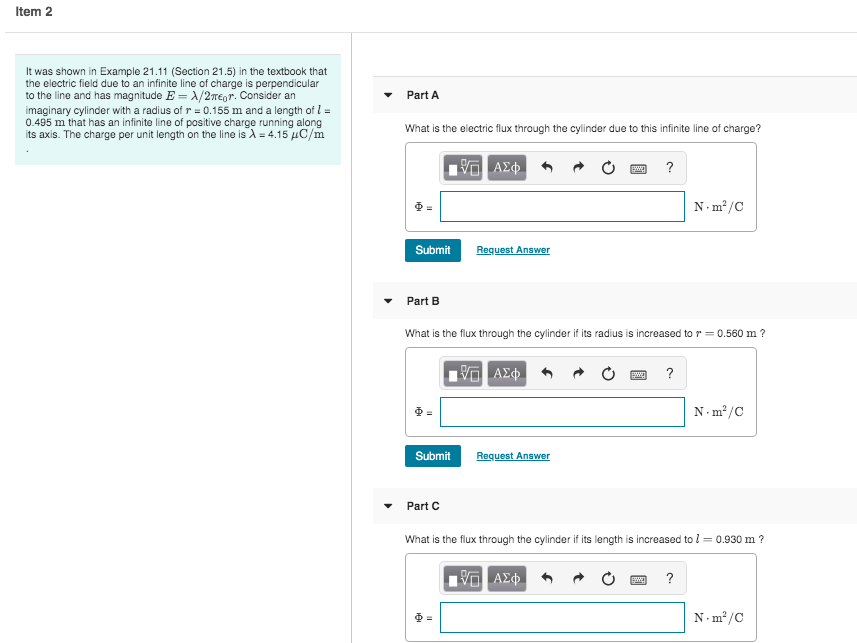Home / Answered Questions / Other / item-2-part-a-it-was-shown-in-example-21-11-section-21-5-in-the-textbook-that-the-electric-field-due-aw812

# (Solved): Item 2 Part A It Was Shown In Example 21.11 (Section 21.5) In The Textbook That The Electric Field D...Item 2 Part A It was shown in Example 21.11 (Section 21.5) in the textbook that the electric field due to an infinite line of charge is perpendicular to the line and has magnitude E= x/2007. Consider an imaginary cylinder with a radius of r = 0.155 m and a length of 1 = 0.495 m that has an infinite line of positive charge running along its axis. The charge per unit length on the line is 2 = 4.15 4C/m What is the electric flux through the cylinder due to this infinite line of charge? VAD O ? N.mÂº/C Submit Request Answer Part B What is the flux through the cylinder if its radius is increased to r=0.560 m ? IVO A NÂ·mÂº/C Submit Request Answer Part C What is the flux through the cylinder if its length is increased to l = 0.930 m ? VO AD O ? Nm"/C

We have an Answer from Expert